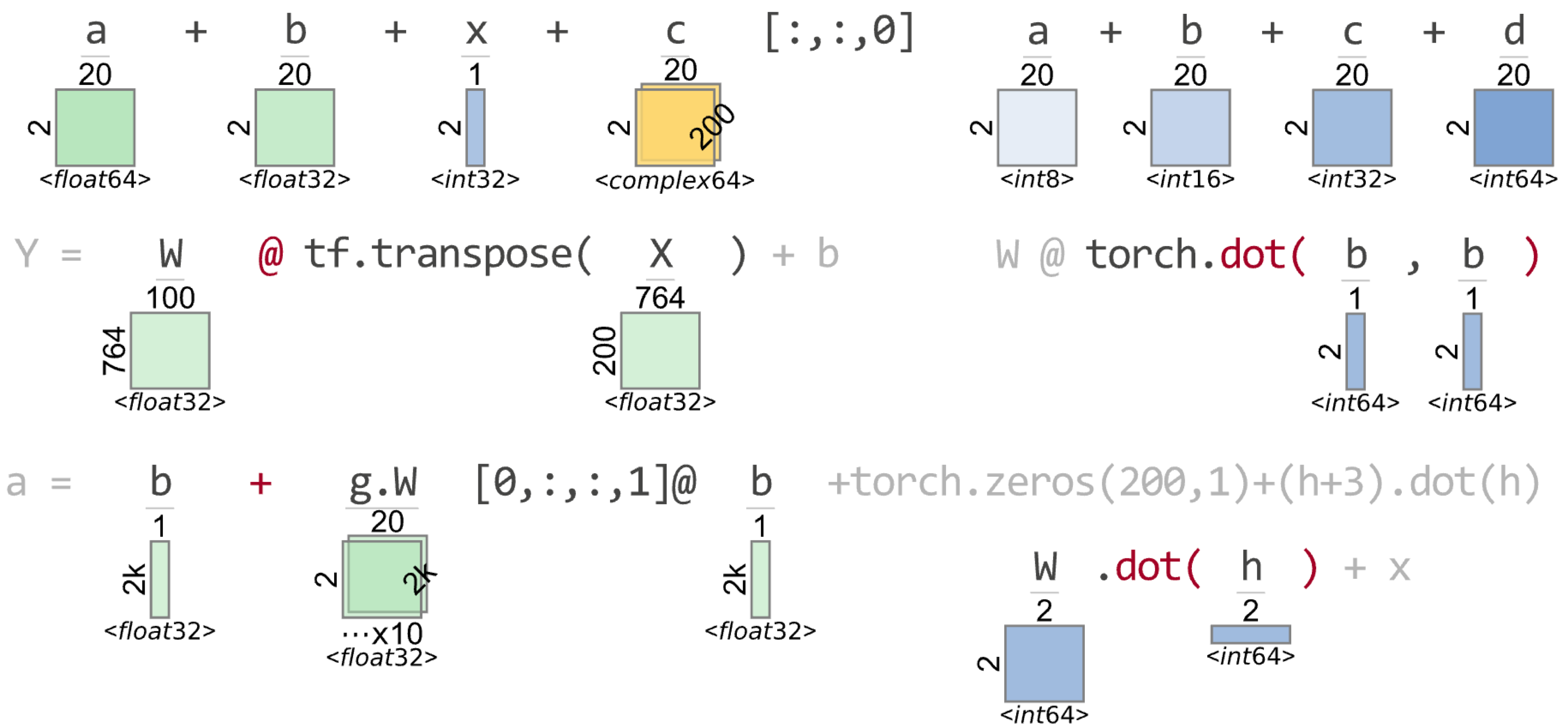# Tensor Sensor

The goal of this library is to generate more helpful exception messages for matrix algebra expressions for numpy, pytorch, jax, tensorflow, keras, fastai.
Alternatives To Tensor Sensor
Transformers112,231641,86910 hours ago114July 18, 2023816apache-2.0Python
🤗 Transformers: State-of-the-art Machine Learning for Pytorch, TensorFlow, and JAX.
Real Time Voice Cloning42,864
2 months ago148otherPython
Clone a voice in 5 seconds to generate arbitrary speech in real-time
Ray27,7138029814 hours ago87July 24, 20233,410apache-2.0Python
Ray is a unified framework for scaling AI and Python applications. Ray consists of a core distributed runtime and a set of AI Libraries for accelerating ML workloads.
Netron24,033469a day ago587August 01, 202323mitJavaScript
Visualizer for neural network, deep learning, and machine learning models
D2l En18,967
21 days ago2November 13, 202295otherPython
Interactive deep learning book with multi-framework code, math, and discussions. Adopted at 500 universities from 70 countries including Stanford, MIT, Harvard, and Cambridge.
Ncnn17,950112 hours ago24November 28, 20221,041otherC++
ncnn is a high-performance neural network inference framework optimized for the mobile platform
Datasets17,1579540a day ago69July 31, 2023601apache-2.0Python
🤗 The largest hub of ready-to-use datasets for ML models with fast, easy-to-use and efficient data manipulation tools
Deeplearning Models15,594
7 months ago5mitJupyter Notebook
A collection of various deep learning architectures, models, and tips
Onnx15,55414839410 hours ago29May 04, 2023320apache-2.0Python
Open standard for machine learning interoperability
Best Of Ml Python14,459
a day ago18cc-by-sa-4.0
🏆 A ranked list of awesome machine learning Python libraries. Updated weekly.
Alternatives To Tensor Sensor
Select To Compare

Alternative Project Comparisons

# Tensor Sensor

(As of September 2021, M1 macs experience illegal instructions in many of the tensor libraries installed via Anaconda, so you should expect TensorSensor to work only on Intel-based Macs at the moment. PyTorch appears to work.)One of the biggest challenges when writing code to implement deep learning networks, particularly for us newbies, is getting all of the tensor (matrix and vector) dimensions to line up properly. It's really easy to lose track of tensor dimensionality in complicated expressions involving multiple tensors and tensor operations. Even when just feeding data into predefined Tensorflow network layers, we still need to get the dimensions right. When you ask for improper computations, you're going to run into some less than helpful exception messages.

To help myself and other programmers debug tensor code, I built this library. TensorSensor clarifies exceptions by augmenting messages and visualizing Python code to indicate the shape of tensor variables (see figure to the right for a teaser). It works with Tensorflow, PyTorch, JAX, and Numpy, as well as higher-level libraries like Keras and fastai.

TensorSensor is currently at 1.0 (December 2021).

## Visualizations

For more, see examples.ipynb at colab. (The github rendering does not show images for some reason: examples.ipynb.)

``````import numpy as np

n = 200         # number of instances
d = 764         # number of instance features
n_neurons = 100 # how many neurons in this layer?

W = np.random.rand(d,n_neurons)
b = np.random.rand(n_neurons,1)
X = np.random.rand(n,d)
with tsensor.clarify() as c:
Y = W @ X.T + b
``````

Displays this in a jupyter notebook or separate window:Instead of the following default exception message:

``````ValueError: matmul: Input operand 1 has a mismatch in its core dimension 0, with gufunc signature (n?,k),(k,m?)->(n?,m?) (size 764 is different from 100)
``````

TensorSensor augments the message with more information about which operator caused the problem and includes the shape of the operands:

``````Cause: @ on tensor operand W w/shape (764, 100) and operand X.T w/shape (764, 200)
``````

You can also get the full computation graph for an expression that includes all of the sub-expression shapes.

``````W = torch.rand(size=(2000,2000), dtype=torch.float64)
b = torch.rand(size=(2000,1), dtype=torch.float64)
h = torch.zeros(size=(1_000_000,), dtype=int)
x = torch.rand(size=(2000,1))
z = torch.rand(size=(2000,1), dtype=torch.complex64)

tsensor.astviz("b = W@b + (h+3).dot(h) + z", sys._getframe())
``````

yields the following abstract syntax tree with shapes:## Install

``````pip install tensor-sensor             # This will only install the library for you
pip install tensor-sensor[torch]      # install pytorch related dependency
pip install tensor-sensor[tensorflow] # install tensorflow related dependency
pip install tensor-sensor[jax]        # install jax, jaxlib
pip install tensor-sensor[all]        # install tensorflow, pytorch, jax
``````

which gives you module `tsensor`. I developed and tested with the following versions

``````\$ pip list | grep -i flow
tensorflow                         2.5.0
tensorflow-estimator               2.5.0
\$ pip list | grep -i numpy
numpy                              1.19.5
numpydoc                           1.1.0
\$ pip list | grep -i torch
torch                              1.10.0
torchvision                        0.10.0
\$ pip list | grep -i jax
jax                                0.2.20
jaxlib                             0.1.71
``````

### Graphviz for tsensor.astviz()

For displaying abstract syntax trees (ASTs) with `tsensor.astviz(...)`, you need the `dot` executable from graphviz, not just the python library.

On Mac, do this before or after tensor-sensor install:

``````brew install graphviz
``````

On Windows, apparently you need

``````conda install python-graphviz  # Do this first; get's dot executable and py lib
pip install tensor-sensor      # Or one of the other installs
``````

## Limitations

I rely on parsing lines that are assignments or expressions only so the clarify and explain routines do not handle methods expressed like:

``````def bar(): b + x * 3
``````

``````def bar():
b + x * 3
``````

watch out for side effects! I don't do assignments, but any functions you call with side effects will be done while I reevaluate statements.

Can't handle `\` continuations.

With Python `threading` package, don't use multiple threads calling clarify(). `multiprocessing` package should be fine.

Also note: I've built my own parser to handle just the assignments / expressions tsensor can handle.

## Deploy (parrt's use)

``````\$ python setup.py sdist upload
``````

``````\$ cd ~/github/tensor-sensor
\$ pip install .
``````

### TODO

• can i call pyviz in debugger?
Popular Tensorflow Projects
Popular Pytorch Projects
Popular Machine Learning Categories
Related Searches

Get A Weekly Email With Trending Projects For These Categories
No Spam. Unsubscribe easily at any time.
Python
Jupyter Notebook
Deep Learning
Pytorch
Tensorflow
Sensor
Matrix
Vector
Numpy
Shape
Tracing
Tensor